Solving One-Step Inequalities MathBitsNotebook.com Terms of Use   Contact Person: Donna Roberts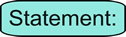If you can solve a linear equation, you can solve a linear inequality. The process is the same, with one exception ...
 ... when you multiply (or divide) an inequality by a negative value, you must change the direction of the inequality.While you should be aware of the exception mentioned above, this web page on one-step inequalities will NOT be multiplying or dividing by negative values.

Let's compare solving an equation with solving an inequality:

The process of adding or subtracting to solve for the variable x is the same when solving equations and inequalities.

 Equation: Solve for x: x + 3 = 5 Solution: Subtract 3 from both sides.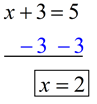Inequality: Solve for x: x + 3 < 5 Solution: Subtract 3 from both sides.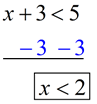The process of multiplying or dividing to solve for the variable x is the same when solving equations and inequalities, for multiplying or dividing by a positive value.
(The "exception" stated above would come into play if multiplying or dividing by a negative value.)

 Equation: Solve for x: 5x = 35 Solution: Divide both sides by 5.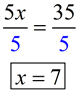Inequality: Solve for x: 5x > 35 Solution: Divide both sides by 5.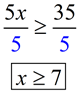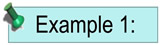Solve for x:     x - 6 > 21 HINT Add 6 to both sides. ANSWER x > 27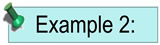Solve for x: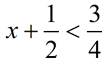HINT Subtract ½ from both sides. Get a common denominator. ANSWER x < ¼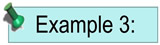Solve for x:    12x < 36 HINT Divide both sides by 12. ANSWER x ≤ 3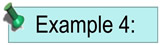Solve for x: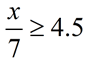HINT Multiply both sides by 7. ANSWER x ≥ 31.5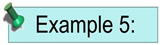Given the inequality, 8x < 24, which choice represents the values that will make this inequality true? A x > 3        B x < 3        C x < 16        D x > -3 HINT Solve the inequality by dividing both sides by 8. ANSWER Choice B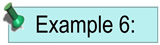What number is not part of the solution set to the inequality b - 12 < 23? A 18        B 24        C 34        D 36 HINT Solve the inequality by adding 12 to both sides. Or just substitute the values into the existing inequality. ANSWER Choice D